Classical Mechanics

# Torque: Level 2-4 Challenges

A frictionless, hollow hemisphere of radius $a$ centered at $O$ is fixed so that its rim is in a horizontal plane. A smooth, uniform rod $AB$ of mass $m$ and length $l$ is in equilibrium, with one end $A$ resting on the inside of the hemisphere and the point $C$ on the rod being in contact with the rim of the hemisphere. The rod is inclined at an angle of $\theta$ with the horizontal, as shown in the diagram.

What is the length of the rod $l$ in terms of $a?$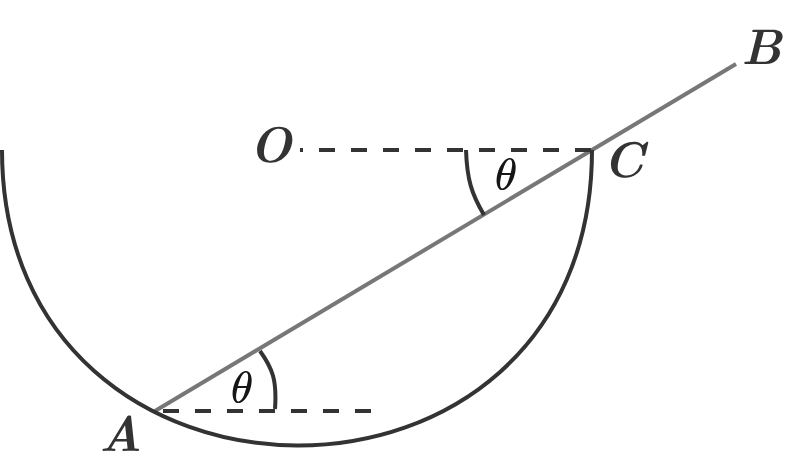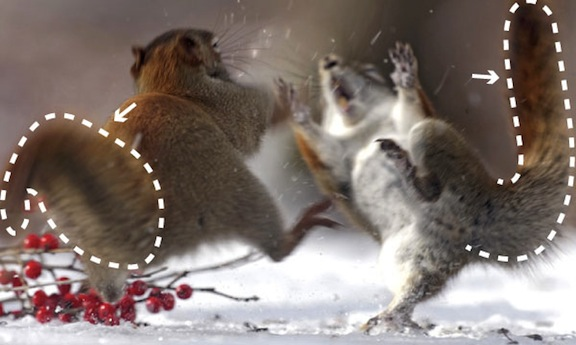These fighting squirrels use their tails: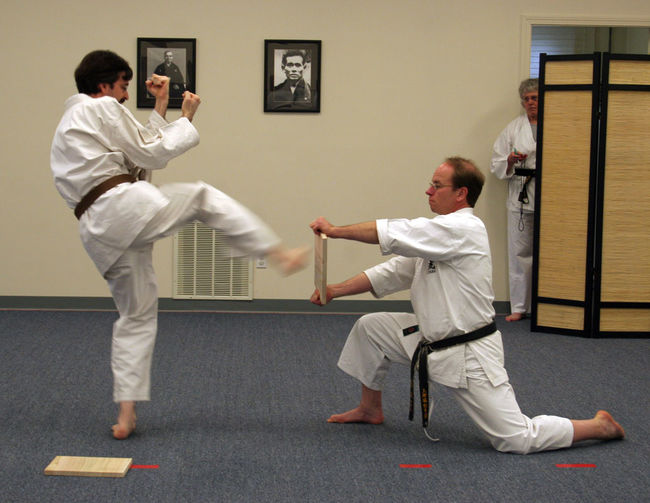Is it easier for the person on the left to break a uniform wooden board of length $l$, which has a mass $m$ than to break a uniform wooden board of twice the length $2l$ and twice the mass $2m$?

Note: Both are equivalent in thickness, density, etc. The person doing the kicking contacts the center of the boards in both cases. Assume that, if the board breaks, it breaks almost immediately after the collision.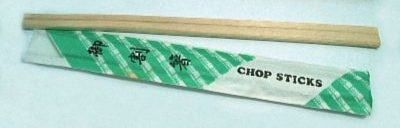Jake is on vacation in Japan (for real!), preparing to enjoy Japanese cuisine when he realizes that breaking chopsticks from the end is far easier than from the middle.

What concept explains this?A perfectly symmetrical tree has a trunk in the shape of a cylinder with diameter $0.2~\mbox{m}$. Since the tree is symmetrical the center of mass is at some distance $L$ above the ground along the axis of the cylinder. A rotten lumberjack with no concept that he's cutting down the most amazing tree ever grown uses a chain saw to cut the tree down. He saws horizontally right at the base of the tree (ground level).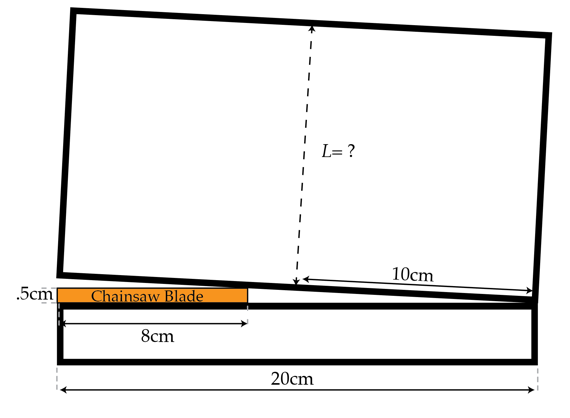What is the minimum value of $L$ in meters such that the tree begins to topple over as soon once the chain saw reaches the position in the picture?

Details and assumptions

• The chain saw blade is $8~\mbox{cm}$ wide and $0.5~\mbox{cm}$ high.
• Treat the tree as a rigid body.

A solid uniform cone having height and radius $R$ both, is given an angular velocity $\omega_{0}$ about its symmetry axis without any translational velocity, and its base kept on a surface having coefficient of kinetic friction $\mu_{k}$. Find the the time in seconds after which its stops rotating.

Details and assumptions

• $R = 49 \text{ cm}$
• $\omega_{0} = 20\text{ rad/s}$
• $\mu_{k} = 0.06$
• $g = 9.8\text{ m/s}^2$
×